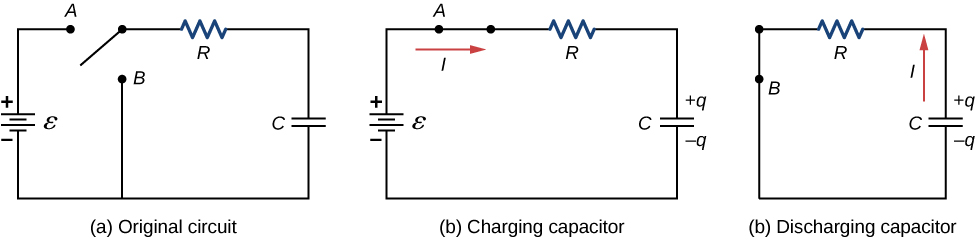# 10.5 Rc circuits

 Page 1 / 5
By the end of the section, you will be able to:
• Describe the charging process of a capacitor
• Describe the discharging process of a capacitor
• List some applications of RC circuits

When you use a flash camera, it takes a few seconds to charge the capacitor that powers the flash. The light flash discharges the capacitor in a tiny fraction of a second. Why does charging take longer than discharging? This question and several other phenomena that involve charging and discharging capacitors are discussed in this module.

## Circuits with resistance and capacitance

An RC circuit    is a circuit containing resistance and capacitance. As presented in Capacitance , the capacitor is an electrical component that stores electric charge, storing energy in an electric field.

[link] (a) shows a simple RC circuit that employs a dc (direct current) voltage source $\epsilon$ , a resistor R , a capacitor C , and a two-position switch. The circuit allows the capacitor to be charged or discharged, depending on the position of the switch. When the switch is moved to position A , the capacitor charges, resulting in the circuit in part (b). When the switch is moved to position B , the capacitor discharges through the resistor.(a) An RC circuit with a two-pole switch that can be used to charge and discharge a capacitor. (b) When the switch is moved to position A , the circuit reduces to a simple series connection of the voltage source, the resistor, the capacitor, and the switch. (c) When the switch is moved to position B , the circuit reduces to a simple series connection of the resistor, the capacitor, and the switch. The voltage source is removed from the circuit.

## Charging a capacitor

We can use Kirchhoff’s loop rule to understand the charging of the capacitor. This results in the equation $\epsilon -{V}_{R}-{V}_{c}=0.$ This equation can be used to model the charge as a function of time as the capacitor charges. Capacitance is defined as $C=q\text{/}V,$ so the voltage across the capacitor is ${V}_{C}=\frac{q}{C}$ . Using Ohm’s law, the potential drop across the resistor is ${V}_{R}=IR$ , and the current is defined as $I=dq\text{/}dt.$

$\begin{array}{c}\epsilon -{V}_{R}-{V}_{c}=0,\hfill \\ \epsilon -IR-\frac{q}{C}=0,\hfill \\ \epsilon -R\frac{dq}{dt}-\frac{q}{C}=0.\hfill \end{array}$

This differential equation can be integrated to find an equation for the charge on the capacitor as a function of time.

$\begin{array}{}\\ \\ \epsilon -R\frac{dq}{dt}-\frac{q}{C}=0,\hfill \\ \frac{dq}{dt}=\frac{\epsilon C-q}{RC},\hfill \\ \underset{0}{\overset{q}{\int }}\frac{dq}{\epsilon C-q}=\frac{1}{RC}\underset{0}{\overset{t}{\int }}dt.\hfill \end{array}$

Let $u=\epsilon C-q$ , then $du=\text{−}dq.$ The result is

$\begin{array}{}\\ \\ \\ \\ \\ -\underset{0}{\overset{q}{\int }}\frac{du}{u}=\frac{1}{RC}\underset{0}{\overset{t}{\int }}dt,\hfill \\ \text{ln}\left(\frac{\epsilon C-q}{\epsilon C}\right)=-\frac{1}{RC}t,\hfill \\ \frac{\epsilon C-q}{\epsilon C}={e}^{\text{−}t}{RC}}.\hfill \end{array}$

Simplifying results in an equation for the charge on the charging capacitor as a function of time:

$q\left(t\right)=C\epsilon \left(1-{e}^{-\frac{t}{RC}}\right)=Q\left(1-{e}^{-\frac{t}{\tau }}\right).$

A graph of the charge on the capacitor versus time is shown in [link] (a). First note that as time approaches infinity, the exponential goes to zero, so the charge approaches the maximum charge $Q=C\epsilon$ and has units of coulombs. The units of RC are seconds, units of time. This quantity is known as the time constant :

$\tau =RC.$

At time $t=\tau =RC$ , the charge is equal to $1-{e}^{-1}=1-0.368=0.632$ of the maximum charge $Q=C\epsilon$ . Notice that the time rate change of the charge is the slope at a point of the charge versus time plot. The slope of the graph is large at time $t=0.0\phantom{\rule{0.2em}{0ex}}\text{s}$ and approaches zero as time increases.

what are waves
In physics, mathematics, and related fields, a wave is a propagating dynamic disturbance (change from equilibrium) of one or more quantities
Discuss how would orient a planar surface of area A in a uniform electric field of magnitude E0 to obtain (a) the maximum flux and (b) the minimum flux through the area.
I'm just doing the first 3 with this message. but thankyou for the time your obviously intending to support us with. viva la accumulation
Marcel
Find the net capacitance of the combination of series and parallel capacitors shown belo
what is ohm?
calculate ideal gas pressure of 0.300mol,v=2L T=40°c
what is principle of superposition
what are questions that are likely to come out during exam
what is electricity
watt is electricity.
electricity ka full definition with formula
Jyoti
If a point charge is released from rest in a uniform electric field will it follow a field line? Will it do so if the electric field is not uniform?
Maxwell's stress tensor is
Yes
doris
neither vector nor scalar
Anil
if 6.0×10^13 electrons are placed on a metal sphere of charge 9.0micro Coulombs, what is the net charge on the sphere
18.51micro Coulombs
ASHOK
Is it possible to find the magnetic field of a circular loop at the centre by using ampere's law?
Is it possible to find the magnetic field of a circular loop at it's centre?
yes
Brother
The density of a gas of relative molecular mass 28 at a certain temperature is 0.90 K kgmcube.The root mean square speed of the gas molecules at that temperature is 602ms.Assuming that the rate of diffusion of a gas in inversely proportional to the square root of its density,calculate the density of
A hot liquid at 80degree Celsius is added to 600g of the same liquid originally at 10 degree Celsius. when the mixture reaches 30 degree Celsius, what will be the total mass of the liquid?
Under which topic
doris
what is electrostatics
Study of charges which are at rest
himanshu

#### Get Jobilize Job Search Mobile App in your pocket Now!By Michael SagBy OpenStaxBy Royalle MooreBy Saylor FoundationBy OpenStaxBy Anh DaoBy Mike WolfBy OpenStaxBy OpenStaxBy OpenStax